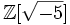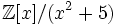# Unique factorization domain

This article defines a property of integral domains, viz., a property that, given any integral domain, is either true or false for that.
View other properties of integral domains | View all properties of commutative unital rings
VIEW RELATED: Commutative unital ring property implications | Commutative unital ring property non-implications |Commutative unital ring metaproperty satisfactions | Commutative unital ring metaproperty dissatisfactions | Commutative unital ring property satisfactions | Commutative unital ring property dissatisfactions

## Definition

### Symbol-free definition

An integral domain is termed a unique factorization domain or factorial domain if every element can be expressed as a product of finite length of irreducible elements (possibly with multiplicity) in a manner that is unique upto the ordering of the elements.

### Definition with symbols

Fill this in later

## Metaproperties

### Polynomial-closedness

This property of integral domains is closed under taking polynomials, i.e., whenever an integral domain has this property, so does the polynomial ring in one variable over it.
View other polynomial-closed properties of integral domains OR view polynomial-closed properties of commutative unital rings

For full proof, refer: Unique factorization is polynomial-closed

### Closure under taking localizations

This property of integral domains is closed under taking localizations: the localization at a multiplicatively closed subset of a commutative unital ring with this property, also has this property. In particular, the localization at a prime ideal, and the localization at a maximal ideal, have the property.
View other localization-closed properties of integral domains | View other localization-closed properties of commutative unital rings

Those irreducibles which occur in the saturation of the multiplicatively closed subset that we invert, no longer remain irreducibles; the others continue to remain irreducible.

Further information: Unique factorization is localization-closed

The quotient of a unique factorization domain by a prime ideal need not be a unique factorization domain. For instance, the ring$\mathbb{Z}[\sqrt{-5}]$, which is not a unique factorization domain, is the quotient$\mathbb{Z}[x]/(x^2 + 5)$ of a unique factorization domain by a prime ideal. Similarly, the ring of trigonometric polynomials$\R[x,y]/(x^2 + y^2 - 1)$ is not a unique factorization domain.

Further information: Unique factorization is not prime-quotient-closed

### Ring of integer-valued polynomials

The ring of integer-valued polynomials over a unique factorization domain need not be a unique factorization domain. The simplest example is where the base ring is the ring of rational integers. Further information: Ring of integer-valued polynomials over ring of rational integers is not a UFD